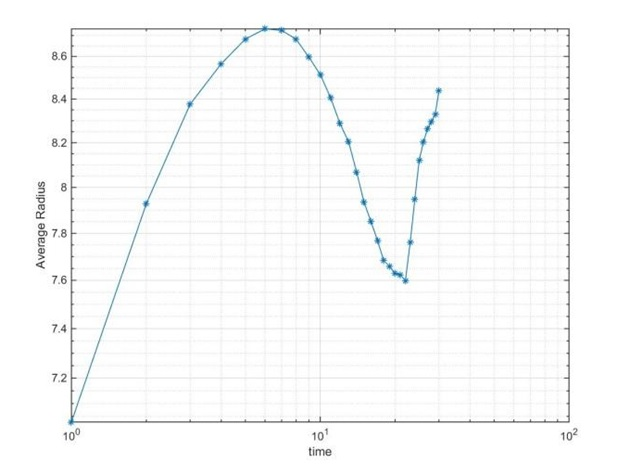• Help with numerical methods assignment on Integration Using Matlab

Help with numerical methods assignment on Integration Using Matlab

The numerical methods assignment helper was tasked with integrating the equation:
${{\frac{\partial_{\rho}}{\partial_t}} ={M{\nabla}^2{\mu}}}$

Assistance with numerical methods homework on Using the Euler scheme.

In this equation, ${{\rho}(\overrightarrow{r},t)}$ – is the density field, ${{\mu}(r,t)}$ – is the chemical potential, and the Laplace operator for 2D case rewrites in the following way:
${{\nabla^2f}={\frac{\partial^2f}{\partial{x^2}}+{\frac{\partial^2f}{\partial{y^2}}}}}$
To find this differentiation, the numerical method homework helper used a second-order central difference scheme in the way:
${{\nabla^2f(i,j)}={\frac{f(i+1j)-2f(ij)=f(i-1j)}{h}+{\frac{f(ij+1)-2f(ij)+f(ij-1)}{h^2}}}}$
For ${\mu(r)}$ the next relation was used:
${{\mu}={RT(1+1nln({\frac{\rho}{1-b\rho}})+{\frac{b\rho}{1-b\rho})}-2a\rho-k\nabla^2\rho}}$
Where for calculation ${\nabla^2\rho}$ the scheme (3) was used.
So, for the Euler scheme we have:
${{\rho(i,j,t+dt)}={\rho(i,j,t)+dt\nabla^2\mu}}$

Online numerical methods tutors getting the finite-differences

In the finite-difference scheme, the online numerical methods tutor used the periodic boundary conditions and the next values for constants and parameters:
${\Delta t = 0.01, \Delta x = \Delta y=1.0, L_x=80, M=10, a=\frac{2}{29}, b=\frac{2}{21}, R=1, T_c=\frac{8a}{27bR}, T=0.7T_c, k=0.025}$
For the level curve superimposed to a contour plot of the concentration field and to a vector plot of the gradient of the density field and for the values ${\rho_{min}(t), \rho(t)}$ and Helmholtz energy
${F=\smallint(\rho RT(1+1nln({\frac{\rho}{1-b\rho }))-a\rho^2-\frac{k}{2}|\nabla \rho|^2)dV}}$
We got the next results (contour plots presented only for some values of t)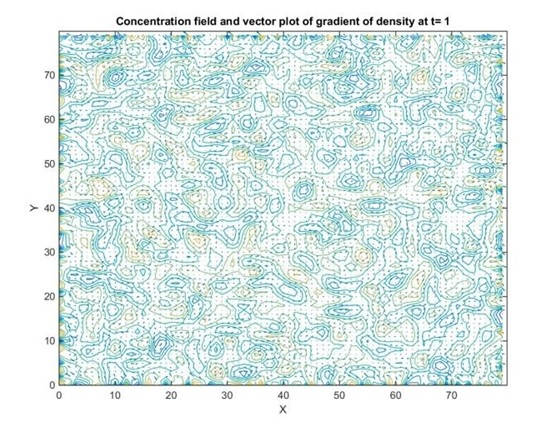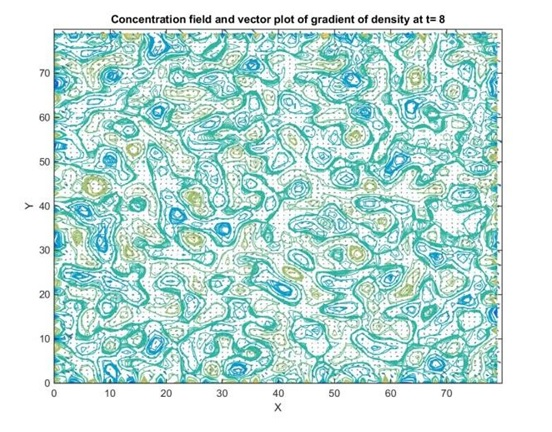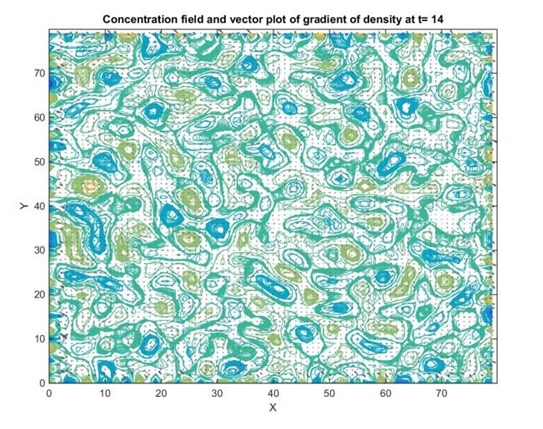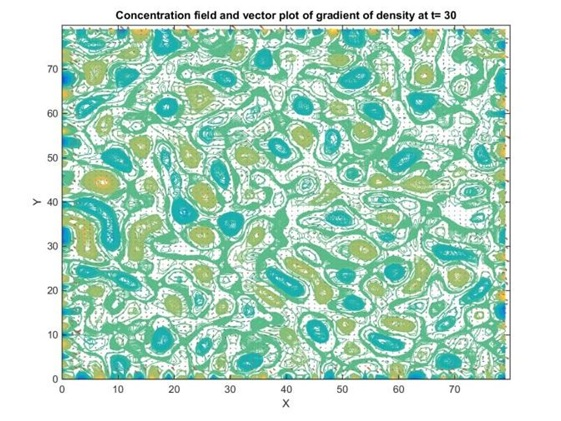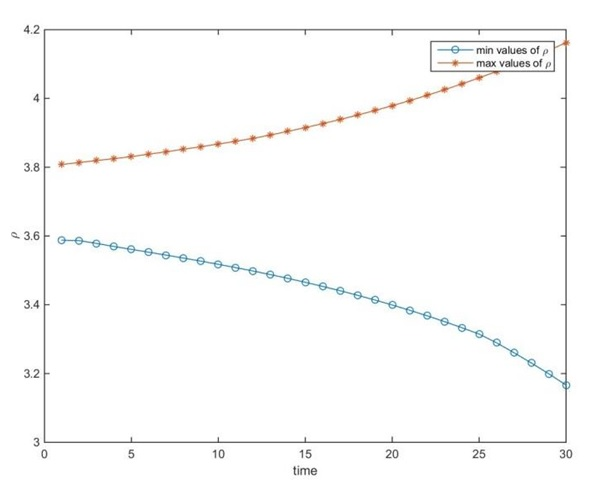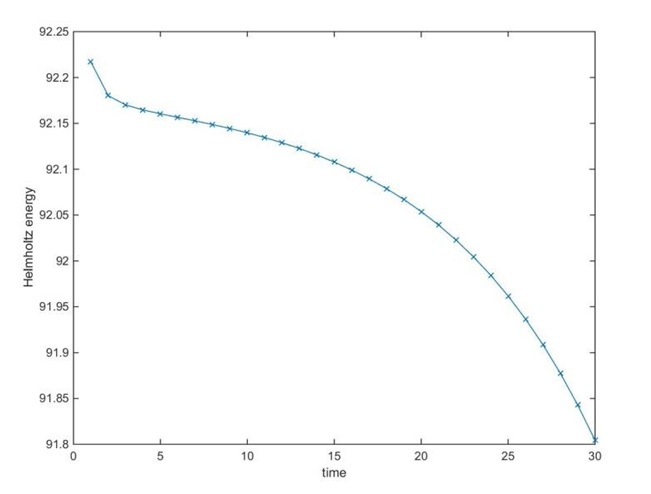Also, in this project, we calculated the radial distribution function of the Fourier transform ${\Omega(q_x,q_y)=F(p(x,y))}$ of the density field as:
${f(|q|)= \frac{\Omega(q)}{\Omega_{tot}}}$
And beneath some results at different times are presented.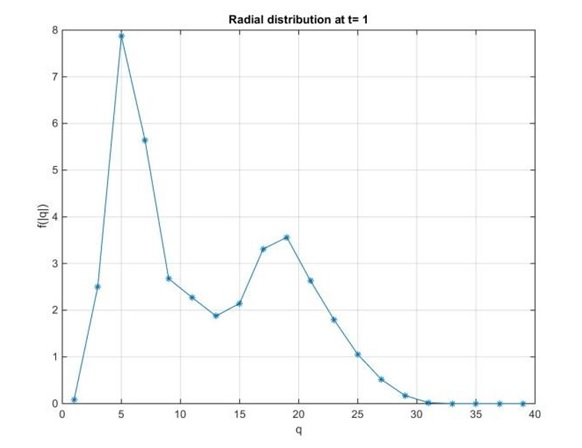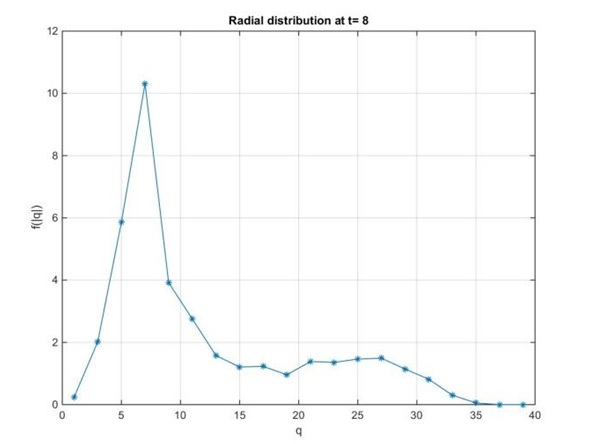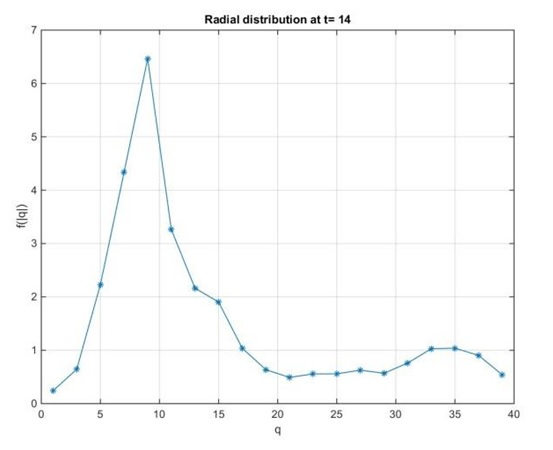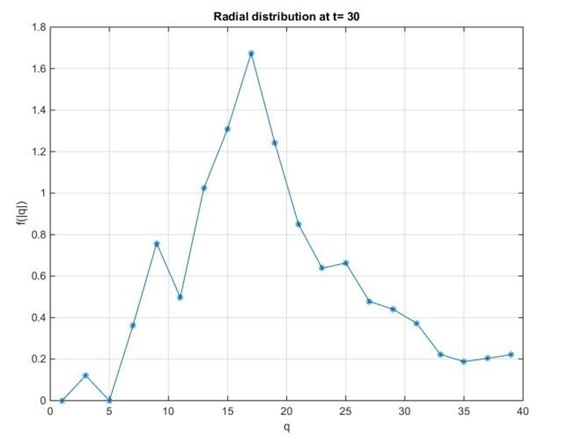These results gave us the possibility to calculate the average size of drops as:
${R^{av}= \frac{L_x \smallint f(q)dq}{\smallint qf(q)dq}}$
In the final part of the project we repeated the above calculations for some different initial densities, these results gave us the possibility to compare the average size as a function of time for initial densities in the following way:
 ${\rho= 2.6-2.8}$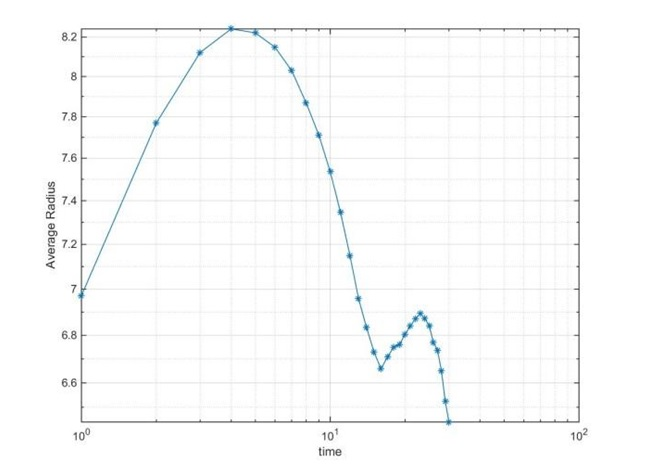${\rho= 3.6-3.8}$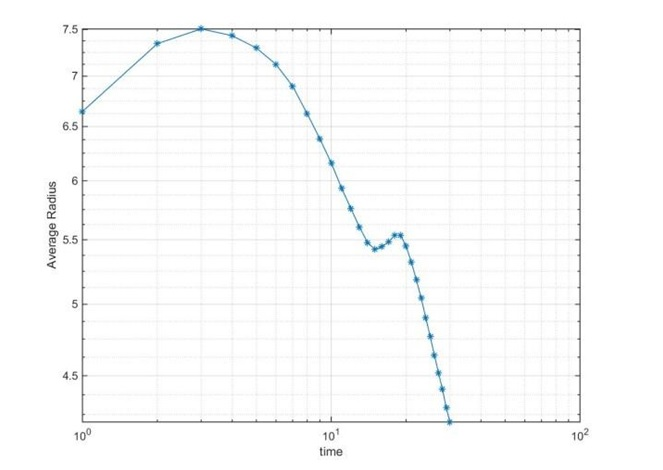${\rho= 4.6-4.8}$